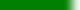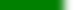# Assessable Learning Goals covered for Quiz 2 Learning GoalsWhat is the difference between a concrete syntax tree and an abstract syntax tree (AST)?Be able to write a non-trivial grammar with recursive rules.Be able to factor out common prefixes in order to make an LL(1) grammar.Given a grammar and its LL(1) parsing table, show how a parse tree is created from a token stream.How are left recursive rules refactored so that a grammar might be processed with an LL(1) parser?How are grammars with common prefixes that create intersecting predict sets refactored to become LL(1) languages?How are top-down parsers that don't use LL tables constructed?How do you build an LL(1) parsing table from a grammar that is LL(1)?How is a predict set calculated from first sets, follow sets, and "derives to λ"?What do the two Ls and the k stand for in "LL(k)" parsing?What does a production rule written with left recursion look like?What is a predict set?What property of it's predict sets make a grammar not LL(1)?Be able to write a non-trivial LL(1) language with recursive rules and operators (precedence and associativity).If a LR(k) parsing table can be formed from a grammar G, what ambiguity conclusion can be made about G?In terms of deciding which production rule to use, what is the fundamental advantage of LR parsers over LL parsers?Understand the shift-reduce parsing algorithm (given a LR parsing table or a simple grammar).What do to the L, R, and k stand for in LR(k) parsing?What type of derivation do "bottum-up" parsers use (generate)?What important property do LR(1) parsers have with respect to the DBL language?What is syntax directed translation (SDT)?Learn how to express logic for parse tree simplification during construction (AKA: syntax directed translation).